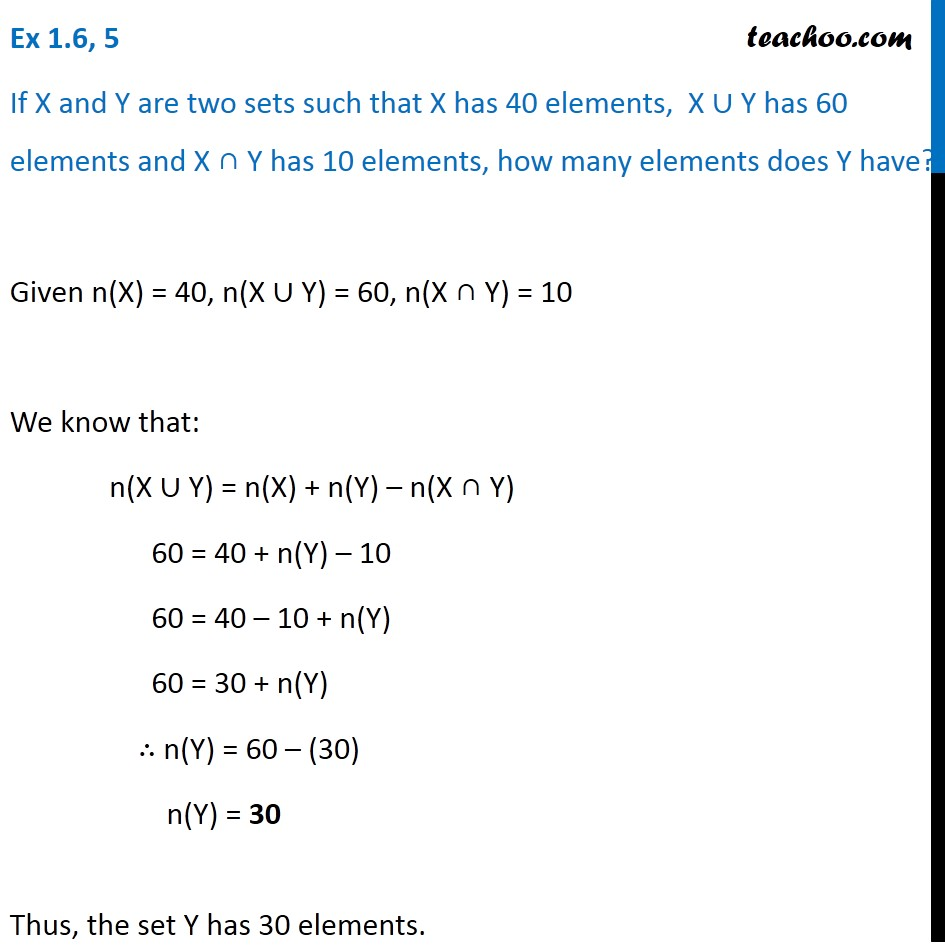Number of elements in set - 2 sets - (Using properties)

Chapter 1 Class 11 Sets
Concept wiseIntroducing your new favourite teacher - Teachoo Black, at only ₹83 per month

### Transcript

Ex 1.6, 5 If X and Y are two sets such that X has 40 elements, X ∪ Y has 60 elements and X ∩ Y has 10 elements, how many elements does Y have? Given n(X) = 40, n(X ∪ Y) = 60, n(X ∩ Y) = 10 We know that: n(X ∪ Y) = n(X) + n(Y) – n(X ∩ Y) 60 = 40 + n(Y) – 10 60 = 40 – 10 + n(Y) 60 = 30 + n(Y) ∴ n(Y) = 60 – (30) n(Y) = 30 Thus, the set Y has 30 elements.